# C++ Program to Find Sum and Average of Two Numbers

In this tutorial you will learn about the C++ Program to Find Sum and Average of Two Numbers and its application with practical example.

In this tutorial, we will learn to create a C++ program that  will Find Sum and Average of Two number  using C++ programming.

## Prerequisites

Before starting with this tutorial we assume that you are best aware of the following C++ programming topics:

• Operators.
• Data type in c++.
• Basic input/output.
• Basic c++ programming.

## C++ Program to Find Sum and Average of Two Numbers

In this program we will find sum and average of two number  given by user . We would first declared and initialized the required variables. Next, we would prompt user to input numbers .Later we will addition and average of numbers.

## Output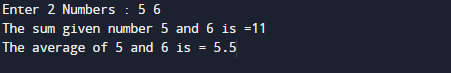In the above program, we have first declared and initialized a set variables required in the program.

• a = it will hold first value.
• b = it will hold second value.
• avg=it will hold average of numbers.

This is one of the basic program in C++ and generally for the understand the basic structure of C++. Here we will  take two numbers as a input from user and calculate it’s addition and  finding average of these two number.

First of all we take  numbers as input from user and store the values in variable a and b respectively.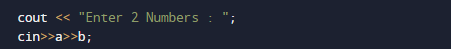And now we will ad these two number add=a+b. and the result store in add variable shown in below image.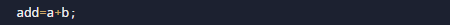After that we will calculate average of these two numbers avg=a+b/2 or avg=add/2.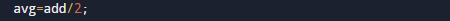In our  program we have taken two numbers as a input from the user and then calculate it’s Addition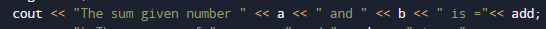and average .In this tutorial we have learn about the C++ Program to Find Sum and Average of Two Numbers and its application with practical example. I hope you will like this tutorial.